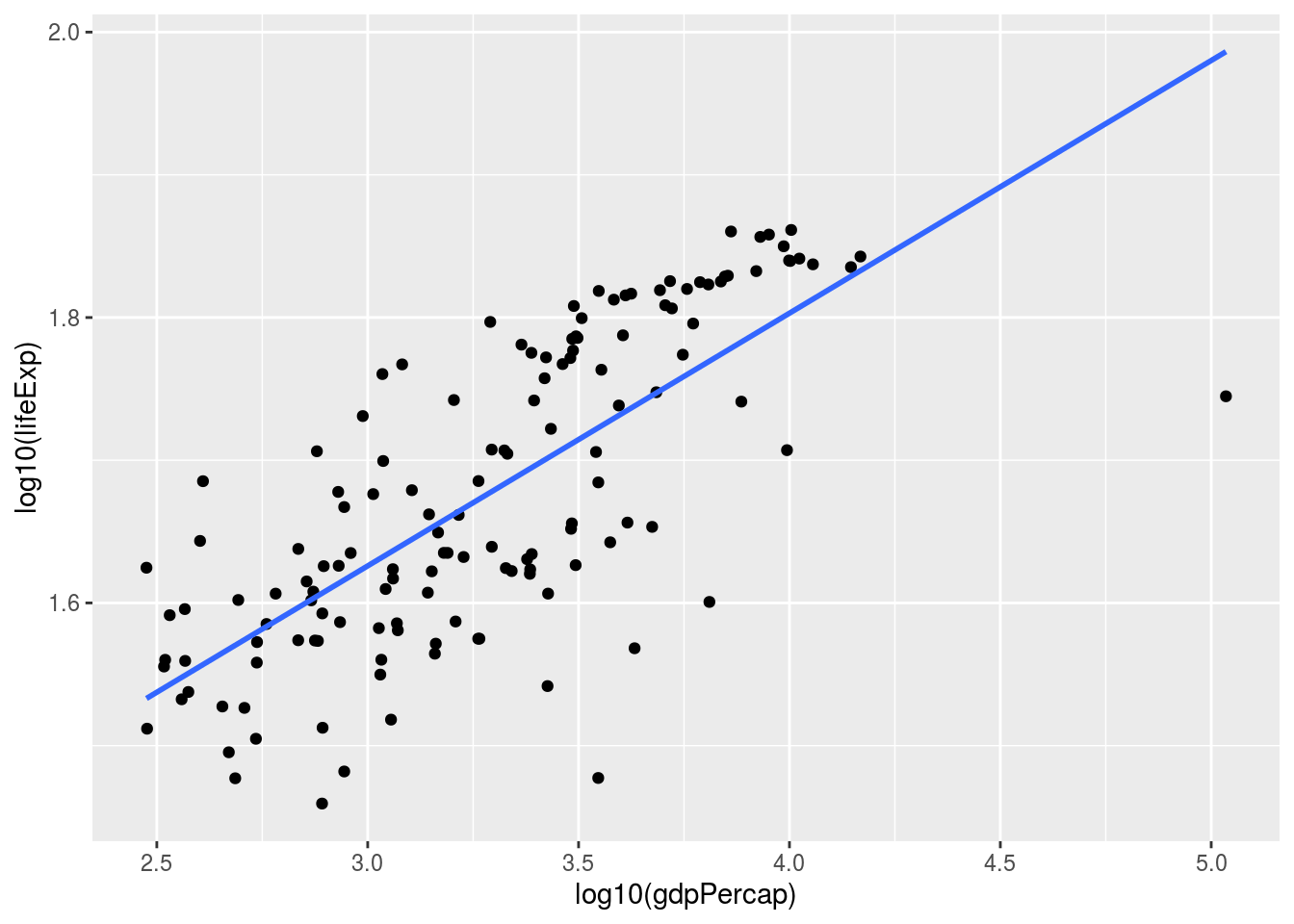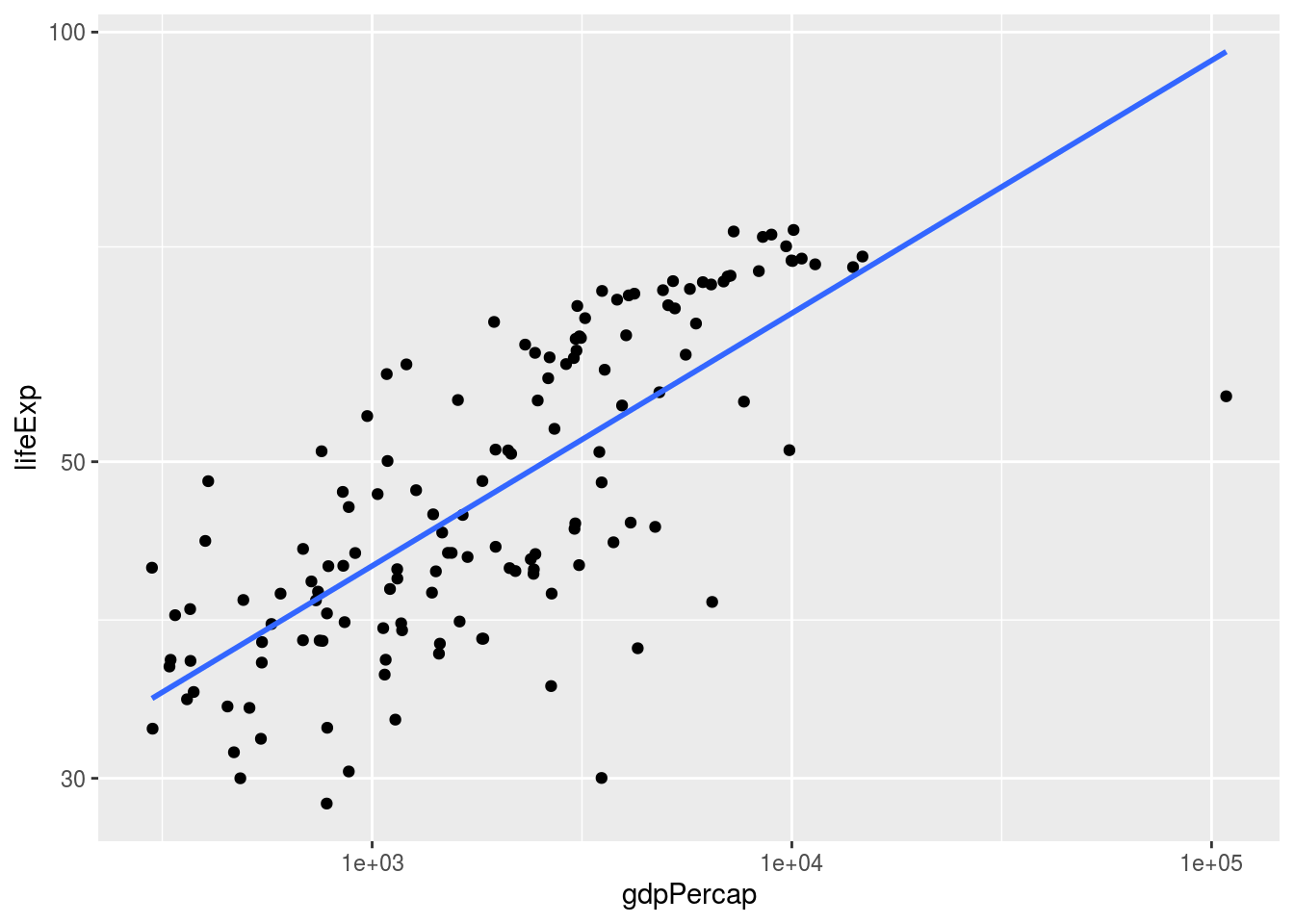Create and save the file p4.R on your computer. The file p3.R, which should contain all the required functions.

Some of you will be tempted to use for and while-loops to solve some of the problems below (if you’ve used those before). Please don’t do this – the goal here is to try to use R the way professional data scientists use it, which usually means no loops.

### Problem 1: Linear Regression

In this problem you will be working with a smaller version of the gapminder dataset. Define

g1952 <- gapminder %>% filter(year == 1952)

#### Problem 1(a)

Plot log10(lifeExp) vs. log10(gdpPercap). Display both a scatterplot and a straight line through the data points. Make sure that you know the syntax for doing this: if you use the lecture notes at first, try to then repeat the same thing without looking at the notes.

##### Solution
g1952 <- gapminder %>% filter(year == 1952)
ggplot(g1952, mapping = aes(x = log10(gdpPercap), y = log10(lifeExp))) +
geom_point() +
geom_smooth(method = "lm", se = F)Another possibility is

ggplot(g1952, mapping = aes(x = gdpPercap, y = lifeExp)) +
geom_point() +
geom_smooth(method = "lm", se = F) +
scale_x_continuous(trans='log10') +
scale_y_continuous(trans='log10')#### Problem 1(b)

Fit a linear regression predicting log(lifeExp) from log(gdpPercap). Fill in the terms in the equation

$\log(\text{lifeExp}) \approx \text{__} + \text{__}\times \log(\text{gdpPercap})$

##### Solution
fit <- lm(log(lifeExp) ~ log(gdpPercap), data = g1952)
fit
##
## Call:
## lm(formula = log(lifeExp) ~ log(gdpPercap), data = g1952)
##
## Coefficients:
##    (Intercept)  log(gdpPercap)
##         2.5207          0.1771

$\log(\text{lifeExp}) \approx 2.5207 + 0.1771\times \log(\text{gdpPercap})$

#### Problem 1(c)

Write a function that takes in a GDP per capita figure, as well the coefficients a_0 and a_1 (such as the ones that you obtained in Problem 1(b)), and returns the predicted life expectancy using those coefficients. Use the facts that $$\exp(\log(z)) = \log(\exp(z)) = z$$ and that if you regressed y on x you can make a prediction using $$\hat{y} = a_0 + a_1 x$$. Your function should be defined using my.pred <- function(a0, a1, gdpPercap). Use this function to estimate the life expectancy in a country with a GDP per capita of $20,000. ##### Solution predict.LE <- function(a0, a1, gdpPercap){ exp(a0 + a1*log(gdpPercap)) } predict.LE(2.5207, 0.1771, 20000) ##  71.85194 #### Problem 1(d) Characterize the relationship between the GDP per capita and the predicted life expectancy. Speicifically, if $$\log(\text{gdpPercap})$$ increases by 1, what is the effect on the predicted $$\log(\text{lifeExp})$$? What is the effect on $$\text{lifeExp}$$? Recall that $$\log(ab) = \log(a) + \log(b)$$, $$\exp\log(z) = \log(\exp(z)) = z$$, and $$\exp(z) = e^z = 2.7^z$$. ##### Solution Suppose we predict $$\log(\text{lifeExp})$$ using $$a_0 + a_1\log(\text{gdpPercap})$$. An increase in $$\log(\text{gdpPercap})$$ by 1 corresponds to an increase by a factor of $$e$$ in $$\text{gdpPercap}$$ because $$\log(\text{gdpPercap}) + 1 = \log(\text{gdpPercap}) + \log\exp(1) = \log(\text{gdpPercap} \times \exp(1)) = \log(\text{gdpPercap} \times e^1) = \log(e\times\text{gdpPercap})$$. Suppose we increase $$\log(\text{gdpPercap})$$ by 1. The new predicted $$\log(\text{lifeExp})$$ increases by $$a_1$$. This corresponds to an increase by a factor of $$e^{a_1}$$ in predicted $$\text{lifeExp}$$. So an increase by a factor of $$e\approx 2.7$$ in $$\text{gdpPercap}$$ corresponds to an increase by a factor of $$e^{a_1} \approx e^0.1771 \approx 1.19$$ in predicted life expectancy. In other words, for a gdpPercap that’s larger by a factor of 2.7, we’d predict a life expectancy that’s higher by about 19%. ### Problem 2 #### Problem 2(a) Write a function which finds the country for which the prediction made by the regression in Problem 1 was the most accurate. Write a function that finds for which country the prediction was the least accurate. Measure the accuracy as the absolute difference between the predicted lifeExp and the actual lifeExp. #### Solution add.pred.errs <- function(g1952){ fit <- lm(log(lifeExp) ~ log(gdpPercap), data = g1952) preds <- exp(predict(fit, newdata = g1952)) g1952 %>% mutate(err = abs(lifeExp - preds)) } most.accurate.pred <- function(g1952){ g1952.errs <- add.pred.errs(g1952) g1952.errs$country[which.min(g1952.errs$err)] } least.accurate.pred <- function(g1952){ g1952.errs <- add.pred.errs(g1952) g1952.errs$country[which.max(g1952.errs$err)] } most.accurate.pred(g1952) ##  Uganda ## 142 Levels: Afghanistan Albania Algeria Angola Argentina Australia ... Zimbabwe least.accurate.pred(g1952) ##  Kuwait ## 142 Levels: Afghanistan Albania Algeria Angola Argentina Australia ... Zimbabwe #### Problem 2(b) Make a dataframe like g1952, but add the column pred.error, which contains the difference between the predicted log(lifeExp) and the actual log(lifeExp). Do you see any patterns? For which countries are we overpredicting and for which countries are we underpredicting? ##### Solution Note that in 2(d), we are looking at the differences between the logs. Arguably we should have continued as in 2(c), but we’ll just go with what the question asked. similarly to 2(c). fit <- lm(log(lifeExp) ~ log(gdpPercap), data = g1952) preds <- predict(fit, newdata = g1952) g1952.err <- g1952 %>% mutate(err = abs(log(lifeExp) - preds)) %>% arrange(desc(err)) g1952.err There is nothing particularly interesting about the countries predicted best – they whole thing about them is that they are (as far as the relationship between GDP per capita and life expectancy) average. Kuwait stands out as a country for which the general trend doesn’t hold. Kuwait started exporting oil around the period of interest. Angola (diamonds) and Saudi Arabia (oil) likewise had their GDP significantly increased due to natural resource exports. ### Problem 3: Approximating Linear Regression In this problem, you will write a function that finds good coefficients for linear regression. #### Problem 3(a): Finding a good coefficient for linear regression Write a function called my.lm that could be used to find the coefficient of Simple Linear Regression. The function could be used like this my.data <- data.frame(X = c(1, 2, 3), Y = c(3.1, 4.9, 7.05)) my.lm(my.data, intercept) # Returns approximately 2, since Y ~ 2*X + 1 The function should work as follows. First, generate possible values for the coefficient using seq(-5, 5, 0.1) ##  -5.0 -4.9 -4.8 -4.7 -4.6 -4.5 -4.4 -4.3 -4.2 -4.1 -4.0 -3.9 -3.8 -3.7 -3.6 ##  -3.5 -3.4 -3.3 -3.2 -3.1 -3.0 -2.9 -2.8 -2.7 -2.6 -2.5 -2.4 -2.3 -2.2 -2.1 ##  -2.0 -1.9 -1.8 -1.7 -1.6 -1.5 -1.4 -1.3 -1.2 -1.1 -1.0 -0.9 -0.8 -0.7 -0.6 ##  -0.5 -0.4 -0.3 -0.2 -0.1 0.0 0.1 0.2 0.3 0.4 0.5 0.6 0.7 0.8 0.9 ##  1.0 1.1 1.2 1.3 1.4 1.5 1.6 1.7 1.8 1.9 2.0 2.1 2.2 2.3 2.4 ##  2.5 2.6 2.7 2.8 2.9 3.0 3.1 3.2 3.3 3.4 3.5 3.6 3.7 3.8 3.9 ##  4.0 4.1 4.2 4.3 4.4 4.5 4.6 4.7 4.8 4.9 5.0 Then, of those possible coefficients, find the one that produces the smallest sum of squared errors. Try different inputs, and make sure that the answer you are getting is close to the answer you would expect. Explain to your preceptor how you came up with the different inputs. #### Solution SSE <- function(coef, intercept, data){ sum((data$Y-(intercept+coef*data$X))^2) } my.lm <- function(data, intercept){ coefs <- seq(-5, 5, 0.01) coefs[which.min(sapply(coefs, FUN=SSE, intercept, data))] } my.data <- data.frame(X = c(1, 2, 3), Y = c(3.1, 4.9, 7.05)) my.lm(my.data, 1) ##  2 #### Problem 3(b): Finding both the intercept and the coefficient (challenge) Now, write a function that finds both a good intercept and a good coefficient. Hint: use a modification of the function in 3(a) that returns both the coefficient and the sum of squared errors in produces. Then repeatedly use that function for every possible intercept hypothesis. SSE <- function(a1, a0, X.vec, Y.vec){ sum((Y.vec-(a0 + a1*X.vec))^2) } best.coef <- function(a0, X.vec, Y.vec){ p <- seq(-5, 5, 0.1) p[which.min(sapply(X = p, FUN = SSE, a0 = a0, X.vec = X.vec, Y.vec = Y.vec))] } best.SSE <- function(a0, X.vec, Y.vec){ # The best (smallest) SSE that can be achieved with intercept a0 # For the particular a0, how small of an SSE can I get? possible.a1 <- seq(-5, 5, 0.005) min(sapply(X = possible.a1, FUN = SSE, a0 = a0, X.vec = X.vec, Y.vec = Y.vec)) } my.lm.2 <- function(X.vec, Y.vec){ possible.a0 <- seq(-5, 5, 0.005) a0 <- possible.a0[which.min(sapply(X = possible.a0, FUN = best.SSE, X.vec = X.vec, Y.vec = Y.vec))] # best.SSE(possible.a0, X.vec, Y.vec) # best.SSE(possible.a0, X.vec, Y.vec) # best.SSE(possible.a0, X.vec, Y.vec) # best.SSE(possible.a0, X.vec, Y.vec) a1 <- best.coef(a0, X.vec, Y.vec) c(a0, a1) } my.data <- data.frame(X = c(1, 2, 3), Y = c(3.1, 4.9, 7.05)) my.lm.2(my.data$X, my.data\$Y)
##  1.065 2.000
lm(Y ~ X, data=my.data)
##
## Call:
## lm(formula = Y ~ X, data = my.data)
##
## Coefficients:
## (Intercept)            X
##       1.067        1.975